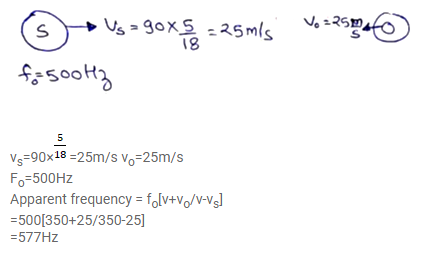# Two trains are travelling towards each other bothQuestion:

Two trains are travelling towards each other both at a speed of $90 \mathrm{~km} \mathrm{~h}^{-1}$. If one of the trains sounds a whistle at $500 \mathrm{~Hz}$, what will be the apparent frequency heard in the other train? Speed of sound in air $=350 \mathrm{~m} \mathrm{~s}^{-1}$.

Solution: